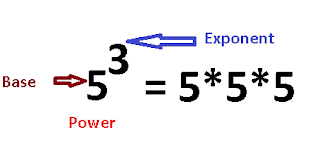# Golang Example - Calculate power or exponents of a Number

In this posts, You will learn Three programs to calculate a power of a number

• First program is to calculate power of a number
• Second program to find exponent of a number using Math pow function
• Third program is to find power of a number using recursive functionPower of m with exponent n is also called m n which is product of number n with m times.
For example, 4 3 is called as 4 to a power of 3
Exponent is 3
Base is 5
Power of 3 base 5 is equal to 5*5*5=125

Power of a number always returns positive number.
The below two programs takes base and exponent input numbers from user using Scanln function, Store this numbers in variables - exponent and base

To understand this example, You should have following features in Go language

### Example program to calculate power of a number using for loop

In this program, Iterated the exponent number using condition exponent != 0
Inside for loop, exponent is multipled with base times and stored the result in variable output
Finally Output is printed to console.
Here is a program to check exponent of a number

``````package main

import (
"fmt"
)

func main() {
var exponent, base int
fmt.Print("Enter Base:")
fmt.Scanln(&base)
fmt.Print("Enter exponent:")
fmt.Scanln(&exponent)

output := 1
for exponent != 0 {
output *= base
exponent -= 1
}
fmt.Printf("Output of power calculation is %d", output)

}

``````

Output is

``````Enter Base:4
Enter exponent:3
Output of power calculation is 64

``````

The above program works only integer numbers of base.
Alternativelly , we can use math pow() function which works with any numbers

### Example program to find exponent of a number using Math pow function

Package math provides mathematical functions. pow() function is used to calcuclate the power of a given exponent and base

Here is the syntax of pow function

``````func Pow(x, y float64) float64

``````

Here is a math pow function example to find power of a number

``````package main
import (
"fmt"
"math"
)

func main() {
var exponent, base float64
fmt.Print("Enter Base:")
fmt.Scanln(&base)
fmt.Print("Enter exponent:")
fmt.Scanln(&exponent)

output := math.Pow(base, exponent)

fmt.Printf("Output of power calculation is %f", output)

}

``````

Output is

``````Enter Base:5
Enter exponent:3
Output of power calculation is 125.000000

``````

### Example program recursive function to calcualte power of a number

Recursive function is a function which call inside a recursive function.

Initally RecurisveFunction is called from a main function for first time

Inside a function It calls until exponent is zero and result is returned from a function

Here is a Recursion function for power of a number

``````package main

import (
"fmt"
)

func RecursivePower(base int, exponent int) int {
if exponent != 0 {
return (base * RecursivePower(base, exponent-1))
} else {
return 1
}
}

func main() {
var exponent, base int
fmt.Print("Enter Base:")
fmt.Scanln(&base)
fmt.Print("Enter exponent:")
fmt.Scanln(&exponent)

output := RecursivePower(base, exponent)

fmt.Printf("Output of power calculation is %d", output)

}

``````
Similar Posts
Subscribe
You'll get a notification every time a post gets published here.

Related posts